# Introduction

This vignette is designed to show the user how the hmma package can be used. This vignette will cover the following subjects:

1. Learn a HMM-A from a data set and using the predict function
2. Create a HMM-A according to a specific specification
3. Use the simulate function to create a data set from a HMM-A

## 1. Create a HMM-A from a data set

First, ensure that you installed the hmma package. Load the package using:

library(hmma)

The hma package contains an example data set called ‘hmmaExampleData’. This data set contains 3 variables (A, B and C) and has 100 observation sequences in which each observation is of length 20. All variable values must be discrete and must be represented as factors in the data set. You can see the contents of this file by typing the name in the console:

hmmaExampleData

The first 5 rows are displayed below:

head(hmmaExampleData$x, 5) ## A B C ## 1 FALSE FALSE FALSE ## 2 FALSE FALSE FALSE ## 3 TRUE FALSE FALSE ## 4 TRUE TRUE FALSE ## 5 TRUE FALSE TRUE The data file must conform to a specific layout: it must be a list where the$x component contains the observations and the $N component is a list with all obersvation lengths. Let us create a HMM-A with 3 states using this data set. To do so, the learnModel function must be used. The function returns a model that fits the data: fit <- learnModel(data = hmmaExampleData, amountOfStates = 3, seed = 1234) To visualise the model, the visualise function can be used: visualise(fit) ## Loading required namespace: Rgraphviz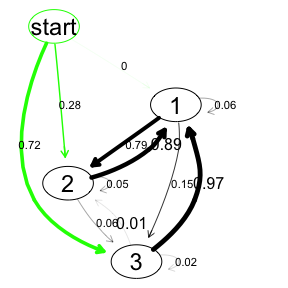The Bayesian networks are not visualised within the states as this would result in unreadable graphs. The Bayesian networks can be visualised using the graphviz.plot method from the bnlearn package. Each state can have a different Bayesian network. The BN for state 1 is visualised and inspected by: library(bnlearn) ## ## Attaching package: 'bnlearn' ## The following object is masked from 'package:stats': ## ## sigma fit$parms.emission[]
##
##   Bayesian network parameters
##
##   Parameters of node A (multinomial distribution)
##
## Conditional probability table:
##  A
##     FALSE      TRUE
## 0.5308398 0.4691602
##
##   Parameters of node B (multinomial distribution)
##
## Conditional probability table:
##
## , , C = FALSE
##
##        A
## B           FALSE      TRUE
##   FALSE 0.6611663 0.3033674
##   TRUE  0.3388337 0.6966326
##
## , , C = TRUE
##
##        A
## B           FALSE      TRUE
##   FALSE 0.8157300 0.4564699
##   TRUE  0.1842700 0.5435301
##
##
##   Parameters of node C (multinomial distribution)
##
## Conditional probability table:
##
##        A
## C           FALSE      TRUE
##   FALSE 0.7229613 0.5804532
##   TRUE  0.2770387 0.4195468
graphviz.plot(fit$parms.emission[])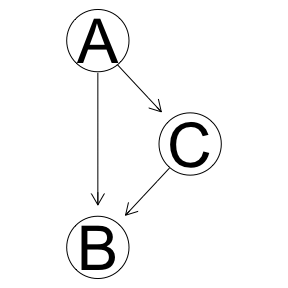We see that an arrow is drawn from A to B but C is unconnected. The other two BNs have a different structure (try them out and verify). Large graphs can become cluttered with low-probability arcs. The visualise method has a parameter minProb that specifies what the minimum probability of an arc must be before it is drawn on the canvas. When we are only interested in arcs with a probability >= 0.10, we call the R code shown below. Note that we have 6 arcs less then the example above. Furthermore, the width of an edge is linearly related to the weight of the edge. It is also possible to use a sigmoid relation between the weight of an edge and its width. Minimum width and maximum width can be specified when calling the visualise method. For more information, please refer to the documentation (?visualise). visualise(fit, minProb = 0.10, minWidth = 0, maxWidth = 5, widthType = 'sigmoid')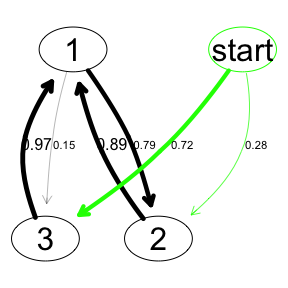### Using a training and validation data set Sometimes we want to use a training set and a validation set. Assume we have the following data sets: training <- list() training$x <- hmmaExampleData$x[1:40,] training$N <- c(20, 20)

validation <- list()
validation$x <- hmmaExampleData$x[41:60,]
validation$N <- c(20) We can now create the model using the training data and check the performance (loglikelihood) using the validation data set: fit2 <- learnModel(training, amountOfStates = 2, seed = 1234) loglikelihood <- predict(fit2, data = validation)$loglik
loglikelihood
##  -61.53877

In this way we can check for multiple states the loglikelihood of the data to the model. A model with a higher loglikelihood better represents the data. This example uses little data, but later in this vignette we will show an example using a larger data set.

### Demonstration of error with unrecognised data

Consider a data file with a variable age that can have three possible values: Y (young), I (intermediate) and O (old). The data set is given by:

obs Age
1 Y
2 Y
3 I
4 I
5 Y
6 I
7 O
8 Y
age <- c("Y", "Y", "I", "I", "Y", "I", "O", "Y")
data <- list()
data$x <- data.frame(age) data$N <- c(8)

The data set is split into a training set and a validation set. The training data set consist out of the first 5 rows:

training <- list()
age <- c("Y", "Y", "I", "I", "Y")
training$x <- data.frame(age, stringsAsFactors = TRUE) training$N <- c(5)

Using this training data set we learn a model just as before:

fit <- learnModel(data = training, amountOfStates = 2, seed = 1234)
visualise(fit)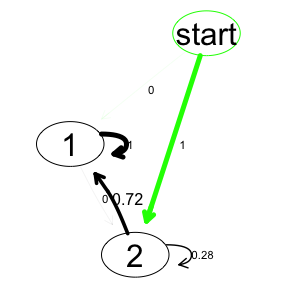Using a validation data set we can check the performance of the model (loglikelihood), assume the validation set contains only the last 3 rows of the complete data set:

validation <- list()
age <- c("I", "O", "Y")
validation$x <- data.frame(age, stringsAsFactors = TRUE) validation$N <- c(3)

To check the performance, we would use the predict function just as before:

loglikelihood <- predict(fit, data = validation)$loglik This however results in an error: “Error in check.data(data): the data set contains Nan/NA values. …”. The reason is as follows: the model is trained with 5 examples that only contain the ages Young and Intermediate. No Old ages were encountered during the training and, consequently, the Bayesian networks that were fitted did not recognise the Old age group during validation. The Bayesian networks in the model can be inspected by: fit$parms.emission[]
##
##   Bayesian network parameters
##
##   Parameters of node age (multinomial distribution)
##
## Conditional probability table:
##  age
##         I         Y
## 0.5528156 0.4471844

This problem can occur with small data sets or data sets that split the values of a variable in many discrete levels. Both increase the probability that a value is not observed in the training data set.

## 2. Create a HMMA via custom specification

Consider the situation in which we would like to create a HMM-A based on a specific specification (and not learned from a data file). For this, we need 3 elements:

1. An initial distribution (probability to start in a state)
2. A transition distribution (the transitions between the states)
3. An emission distribution (the Bayesian networks in the states)

For this example we contruct the HMM-A from the image below.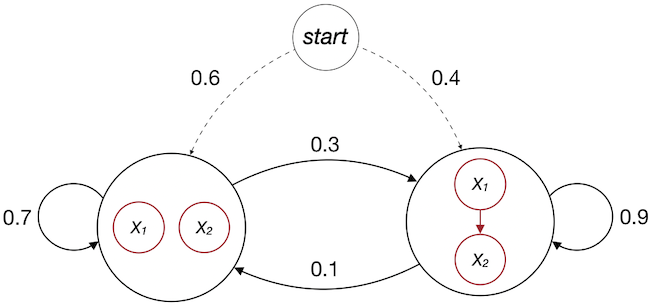A HMM-A

init <- c(0.6, 0.4)
init
##  0.6 0.4

Now we need to specify the transition distribution:

trans <- matrix(c(0.7, 0.3, 0.1, 0.9), nrow = 2, ncol = 2, byrow = TRUE)
trans
##      [,1] [,2]
## [1,]  0.7  0.3
## [2,]  0.1  0.9

Finally, the emission distributions must be specified. Each state has a different Bayesian network. We start with the network from state 1 (the leftmost state) and then create the Bayesian network from state 2. The image above does not show the conditional probability tables and therefore we will assume that X1 and X2 have possible values TRUE and FALSE with a certain probability. To learn more about the creation of Bayesian networks, please refer to the documenation of the bnlearn package (bnlearn website).

library(bnlearn)
struc <- model2network("[X1][X2]")
cptX1 <- matrix(c(0.15, 0.85), ncol = 2, dimnames = list(NULL, c("TRUE", "FALSE")))
cptX2 <- matrix(c(0.7, 0.3), ncol = 2, dimnames = list(NULL, c("TRUE", "FALSE")))

bn1 <- custom.fit(struc, dist = list(X1 = cptX1,
X2 = cptX2))

struc <- model2network("[X2|X1][X1]")
cptX1 <- matrix(c(0.4, 0.6), ncol = 2, dimnames = list(NULL, c("TRUE", "FALSE")))
cptX2 <- matrix(c(0.9, 0.1, 0.5, 0.5), nrow = 2, ncol = 2)
dimnames(cptX2) <- list("X2" = c("TRUE", "FALSE"),
"X1" = c("TRUE", "FALSE"))

bn2 <- custom.fit(struc, dist = list(X1 = cptX1,
X2 = cptX2))

bns <- list()
bns[] <- bn1
bns[] <- bn2

Now it is time for final assembly. We use the function createHmma from the hmma package:

model <- createHmma(init = init, trans = trans, bns = bns)

To visualise the model and the different Bayesian networks within the states:

visualise(model = model)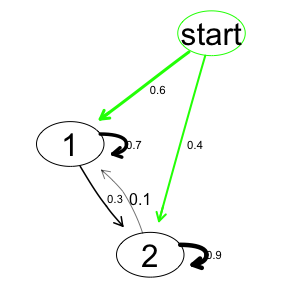graphviz.plot(model$parms.emission[])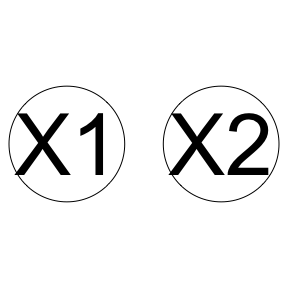graphviz.plot(model$parms.emission[])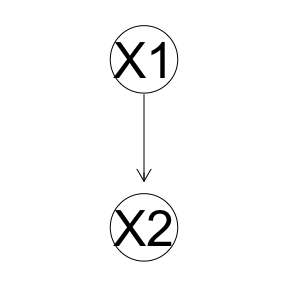## 3. Use the simulate function to create a data set from a HMM-A

A HMM-A model can be used to generate simulated data. This can be done by using the simulate function. We use the model created in chapter 2 of this vignette.

data <- simulate(model, nsim = c(20, 20, 20, 20), seed = 1234)
data
## $s ##  1 1 1 1 2 2 1 1 1 1 1 1 1 2 2 2 2 2 2 2 1 1 1 1 1 2 2 2 2 1 1 1 1 1 1 2 2 2 ##  2 2 1 1 1 1 1 1 1 1 1 2 1 1 2 2 2 2 2 2 2 2 2 1 1 1 1 2 2 2 1 1 1 2 1 2 1 1 ##  1 1 1 1 ## ##$x
##       X1      X2
##  [1,] "TRUE"  "TRUE"
##  [2,] "FALSE" "TRUE"
##  [3,] "FALSE" "FALSE"
##  [4,] "FALSE" "TRUE"
##  [5,] "FALSE" "TRUE"
##  [6,] "FALSE" "TRUE"
##  [7,] "FALSE" "TRUE"
##  [8,] "FALSE" "TRUE"
##  [9,] "FALSE" "TRUE"
## [10,] "FALSE" "FALSE"
## [11,] "FALSE" "TRUE"
## [12,] "FALSE" "TRUE"
## [13,] "FALSE" "TRUE"
## [14,] "FALSE" "FALSE"
## [15,] "FALSE" "FALSE"
## [16,] "TRUE"  "TRUE"
## [17,] "TRUE"  "TRUE"
## [18,] "FALSE" "TRUE"
## [19,] "TRUE"  "TRUE"
## [20,] "FALSE" "TRUE"
## [21,] "FALSE" "FALSE"
## [22,] "TRUE"  "FALSE"
## [23,] "FALSE" "TRUE"
## [24,] "FALSE" "TRUE"
## [25,] "FALSE" "TRUE"
## [26,] "TRUE"  "TRUE"
## [27,] "FALSE" "FALSE"
## [28,] "TRUE"  "TRUE"
## [29,] "TRUE"  "TRUE"
## [30,] "FALSE" "FALSE"
## [31,] "FALSE" "FALSE"
## [32,] "FALSE" "TRUE"
## [33,] "FALSE" "TRUE"
## [34,] "FALSE" "TRUE"
## [35,] "TRUE"  "TRUE"
## [36,] "FALSE" "FALSE"
## [37,] "FALSE" "TRUE"
## [38,] "FALSE" "TRUE"
## [39,] "TRUE"  "FALSE"
## [40,] "FALSE" "TRUE"
## [41,] "FALSE" "TRUE"
## [42,] "FALSE" "TRUE"
## [43,] "FALSE" "TRUE"
## [44,] "FALSE" "TRUE"
## [45,] "FALSE" "TRUE"
## [46,] "FALSE" "TRUE"
## [47,] "FALSE" "TRUE"
## [48,] "FALSE" "FALSE"
## [49,] "FALSE" "TRUE"
## [50,] "FALSE" "FALSE"
## [51,] "FALSE" "TRUE"
## [52,] "FALSE" "TRUE"
## [53,] "TRUE"  "TRUE"
## [54,] "TRUE"  "TRUE"
## [55,] "FALSE" "TRUE"
## [56,] "TRUE"  "FALSE"
## [57,] "FALSE" "TRUE"
## [58,] "TRUE"  "TRUE"
## [59,] "TRUE"  "TRUE"
## [60,] "TRUE"  "TRUE"
## [61,] "TRUE"  "TRUE"
## [62,] "FALSE" "FALSE"
## [63,] "FALSE" "FALSE"
## [64,] "FALSE" "TRUE"
## [65,] "FALSE" "FALSE"
## [66,] "FALSE" "FALSE"
## [67,] "FALSE" "TRUE"
## [68,] "FALSE" "TRUE"
## [69,] "FALSE" "FALSE"
## [70,] "FALSE" "FALSE"
## [71,] "FALSE" "TRUE"
## [72,] "FALSE" "FALSE"
## [73,] "FALSE" "TRUE"
## [74,] "TRUE"  "TRUE"
## [75,] "FALSE" "TRUE"
## [76,] "FALSE" "TRUE"
## [77,] "FALSE" "FALSE"
## [78,] "FALSE" "TRUE"
## [79,] "FALSE" "TRUE"
## [80,] "FALSE" "FALSE"
##
## \$N
##  20 20 20 20
##
## attr(,"class")
##  "mhsmm.data"

The data above consists of 4 seperate observation sequences, each of length 20.